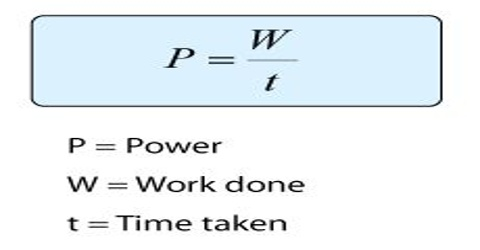# Power Definition in Physics

Power is the time rate of doing work of an agent and it is measured by work done in unit time. Whether displacement of a body due to the action of force has been done quickly or slowly is understood by power, not by the amount of work.

Power is a scalar quantity. It does not depend only on total amount of work done, but on how long it takes to do that work. If time takes to do a particular work is less, then its power is more. Power is the rate at which work is done or energy is transferred in a unit of time. It is increased if work is done faster or energy is transferred in less time.

Sometimes, it is important to know what work is done than the amount of work done. For example, while buying a machine it is not considered what amount of work the machine can do, rather how quickly it can do the work is taken into consideration. Speedily the machine can do work, much better is that engine. Besides, by applying force on a machine or body if there is change of motion then we say that the machine or the body has power to do work.For example, a machine does work of 2000 Joules in 4 hours and another machine does work of 2400 Joules in 6 hours. Then power of the first machine 2000/4 = 500 Joule/hour. Power of the second machine = 2400/6 Joule/hour. So, although amount of work done by the first machine is less than that of the second machine, but power of the first machine is more.